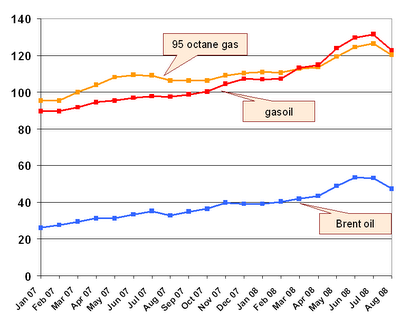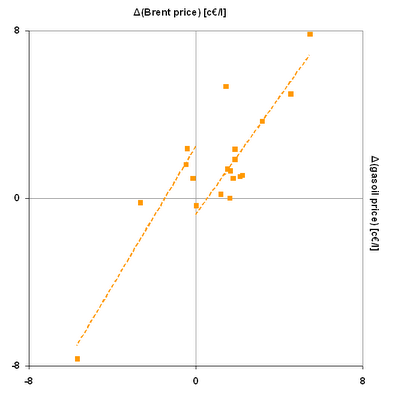## Wednesday, November 12, 2008

### Gas price hysteresis

Due to the extreme rise of gas prices during the last couple of years, car users have become very sensitive (if not specially elastic) to the translation of oil price variations to retail gasoline prices. In particular, some have expressed the suspicion that increases in oil price are rapidly transferred to gas stations, whereas when the oil price decreases, this reflects less directly into a reduction of retail gas prices. Is this a case of actual price hysteresis or just consumer hysteria?

The figure shows the evolution of Brent prices and average retail prices of 95 octane gasoline and gasoil (diesel) in Spain, all in c€/l (source: Cores, via WonkaPistas).The final price of a liter of gasoline or gasoil in Spain can be expressed as

final price = (1 + VAT) × (price before taxes + special tax),

where the applicable VAT is currently 16% and the special tax, known as the impuesto especial sobre hidrocarburos, is approximately fixed. So, the variations on this price do not depend on this special tax:

Δ(final price) = (1 + VAT) × Δ(price before taxes).

These variations can be then attributed to the volatile part of the product cost, the most important of which is oil price. This is how Δ(gasoline price before taxes) plots against Δ(Brent price) monthly between February 2007 and August 2008:The dotted lines are the linear regressions of the points for Δ(Brent price) ≥ 0 and ≤ 0, respectively:

ΔBrent ≥ 0 → y = f+(x) = b+ + m+x = −0.5378 + 0.9885x,
ΔBrent ≤ 0 → y = f(x) = b + mx = 2.4610 + 1.4865x,

How can we interpret this? If the variations of gasoline price were symmetrical with respect to the sign of Δ(Brent price), we would have

f+(x) = −f(−x),
b+ = b,
m+ = m,

which is clearly not the case. In fact, we have that

f+(x) > −f(−x) for x in [0,3.86),

that is, variations in the price of the gasoline when 0 ≤ ΔBrent < 3.86 are larger than the corresponding reductions when − 3.86 < ΔBrent ≤ 0. In this interval, gasoline price can be said to present hysteresis unfavorable to the consumer. The situation is reversed when ΔBrent > 3.86, though this phenomenom is mainly accounted for by the presence of the outlier point at (−5.64,−5.41).

As for the variations on the price of gasoil before taxes, we have the following:with regression lines

ΔBrent ≥ 0 → y = f+(x) = b+ + m+x = −0.7877 + 1.3841x,
ΔBrent ≤ 0 → y = f(x) = b + mx = 2.4676 + 1.6557x,

resunting in an user unfavorable hysteresis interval −6.19 < ΔBrent < 6.19, which spans the entire dataset.

In conclusion, only to some extent does the analysis corroborate the feeling many users share that oil companies do not reflect oil price reductions as faithfully as they do reflect increases. These results are not very trustworthy since negative variations of oil price are underrepresented. Given the recent drop in the international oil market, it will be interesting to repeat the calculations when the data for the last two months become available.